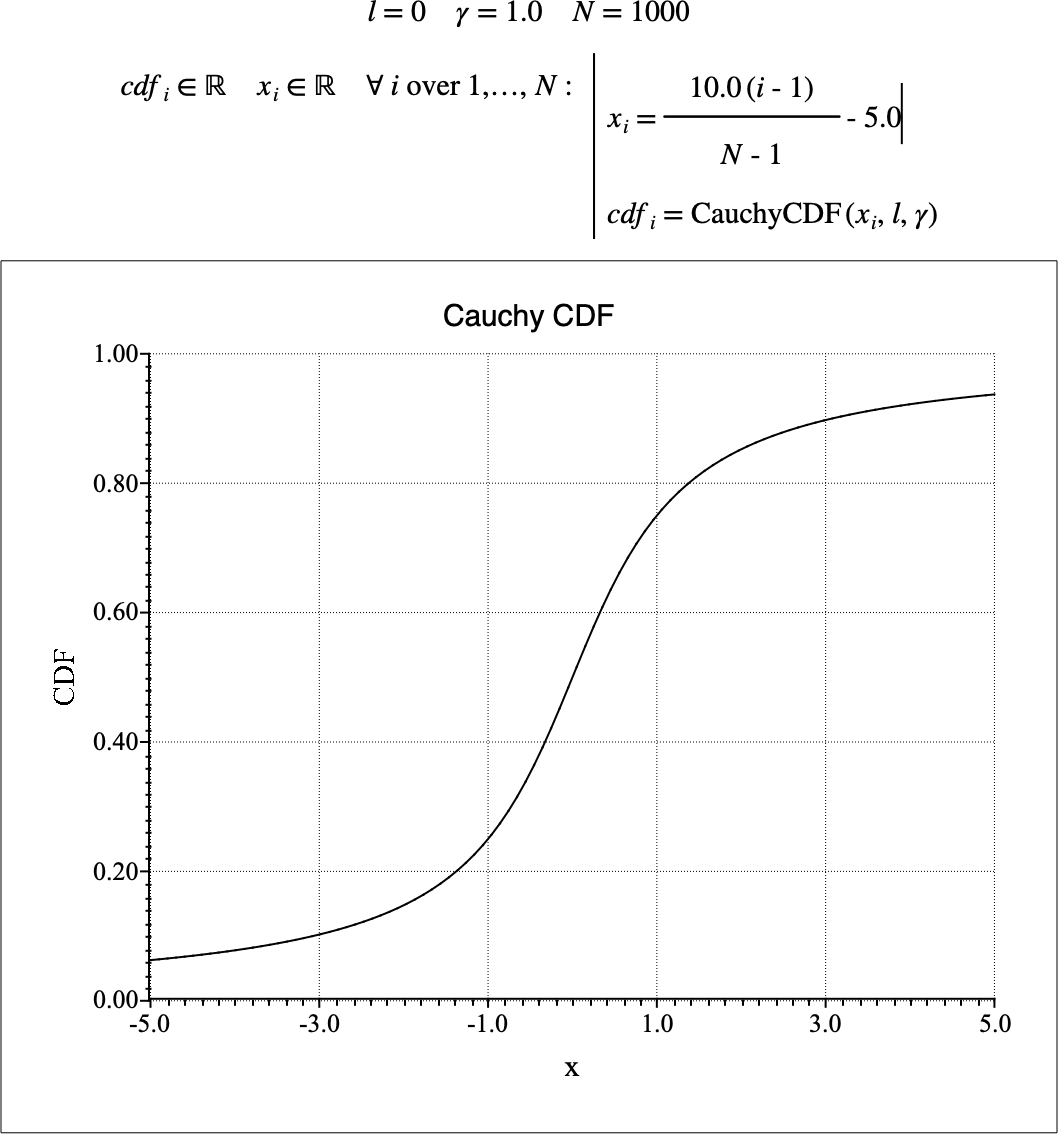# $$\text{CauchyCDF}$$¶

You can use the $$\text{CauchyCDF}$$ function to calculate the cumulative distribution function (CDF) of the Cauchy-Lorentz distribution.

You can use the \cauchyc backslash command to insert this function.

The following variants of this function are available:

• $$\text{real } \text{CauchyCDF} \left ( \text{<x>}, \text{<location>}, \text{<}\gamma\text{>} \right )$$

Where $$x$$, $$location$$, and $$\gamma$$ are scalar values representing the value of interest, the location or offset, and the scale term respectively. Note that this function is defined over the range $$\gamma > 0$$ and will generate a runtime error or return NaN for values for which the function is not defined.

The value is calculated directly using the relation:

$\text{CauchyCDF} \left ( x, l, \gamma \right ) = \frac{1}{\pi} \text{arctan} \left ( \frac{x - l}{\gamma} \right ) + \frac{1}{2}$

Figure 112 shows the basic use of the $$\text{CauchyCDF}$$ function.Figure 112 Example Use Of the CauchyCDF Function